# Certainty Factors 1 Definition A certainty factor cf

• Slides: 18Certainty Factors 1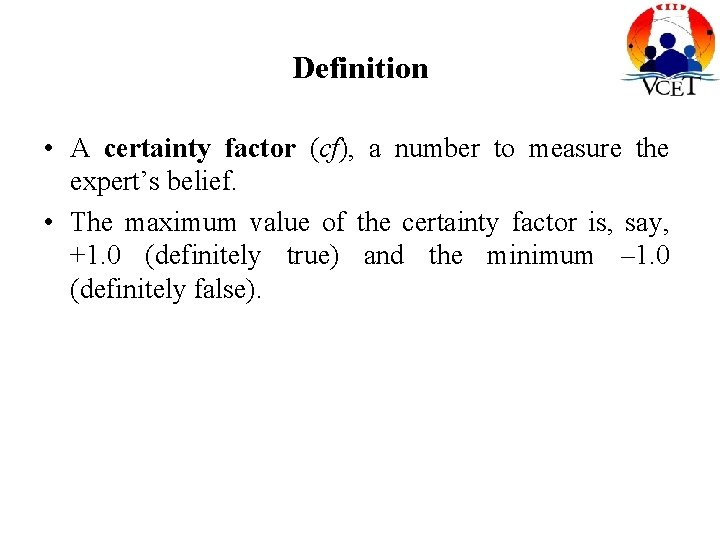Definition • A certainty factor (cf), a number to measure the expert’s belief. • The maximum value of the certainty factor is, say, +1. 0 (definitely true) and the minimum – 1. 0 (definitely false).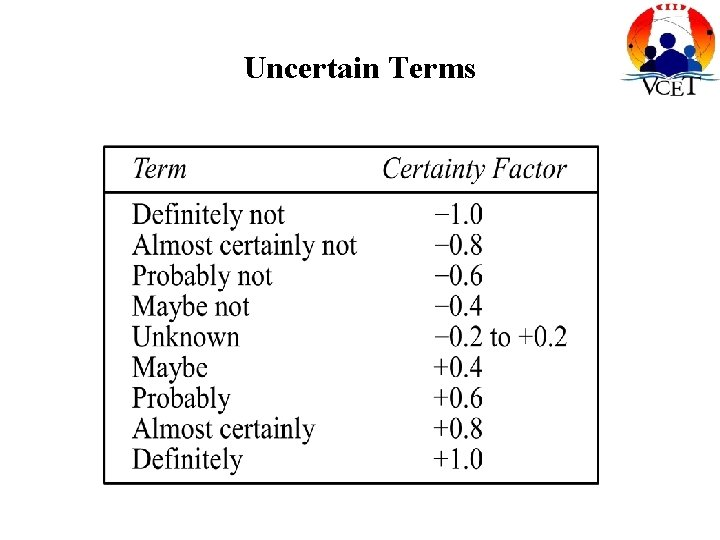Uncertain Terms• For example, if the expert states that some evidence is almost certainly true, a cf value of 0. 8 would be assigned to this evidence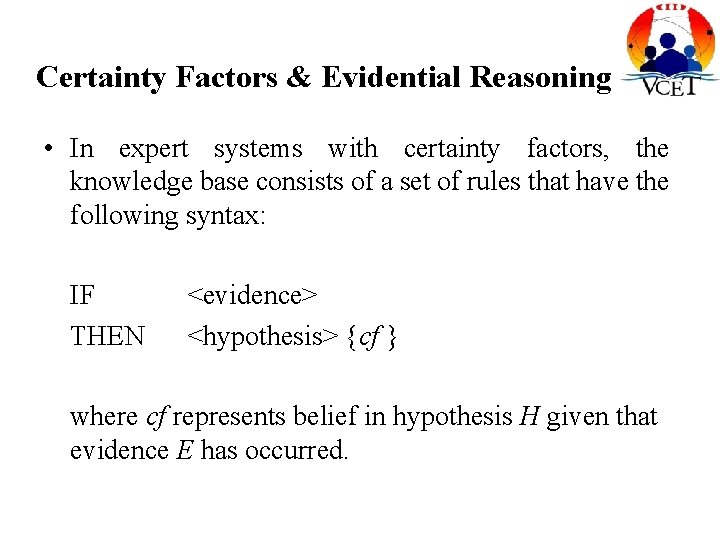Certainty Factors & Evidential Reasoning • In expert systems with certainty factors, the knowledge base consists of a set of rules that have the following syntax: IF THEN <evidence> <hypothesis> {cf } where cf represents belief in hypothesis H given that evidence E has occurred.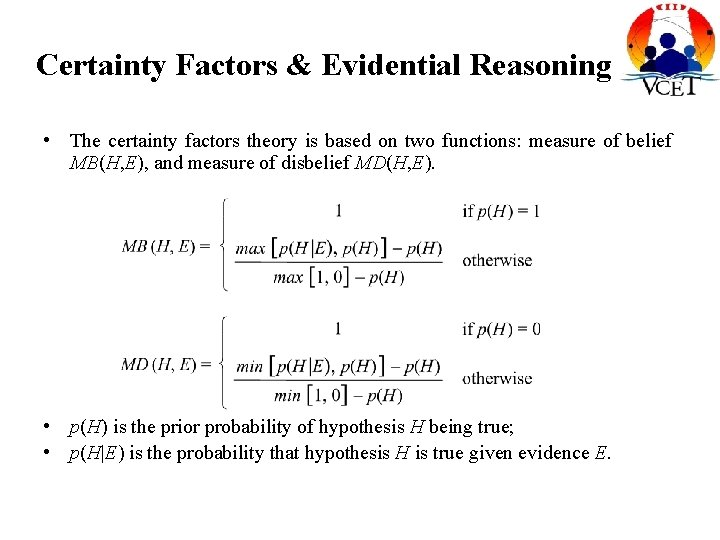Certainty Factors & Evidential Reasoning • The certainty factors theory is based on two functions: measure of belief MB(H, E), and measure of disbelief MD(H, E). • p(H) is the prior probability of hypothesis H being true; • p(H|E) is the probability that hypothesis H is true given evidence E.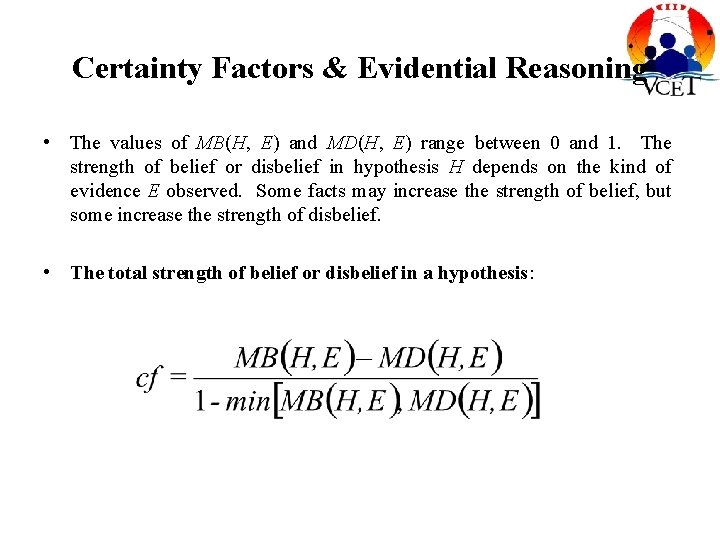Certainty Factors & Evidential Reasoning • The values of MB(H, E) and MD(H, E) range between 0 and 1. The strength of belief or disbelief in hypothesis H depends on the kind of evidence E observed. Some facts may increase the strength of belief, but some increase the strength of disbelief. • The total strength of belief or disbelief in a hypothesis: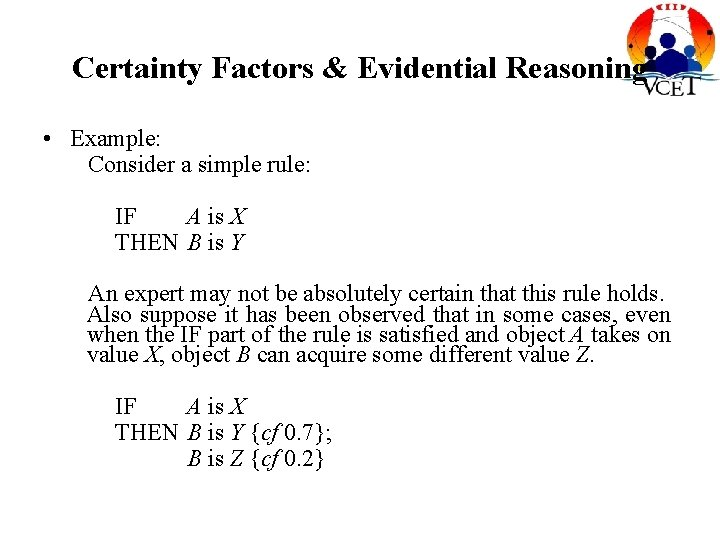Certainty Factors & Evidential Reasoning • Example: Consider a simple rule: IF A is X THEN B is Y An expert may not be absolutely certain that this rule holds. Also suppose it has been observed that in some cases, even when the IF part of the rule is satisfied and object A takes on value X, object B can acquire some different value Z. IF A is X THEN B is Y {cf 0. 7}; B is Z {cf 0. 2}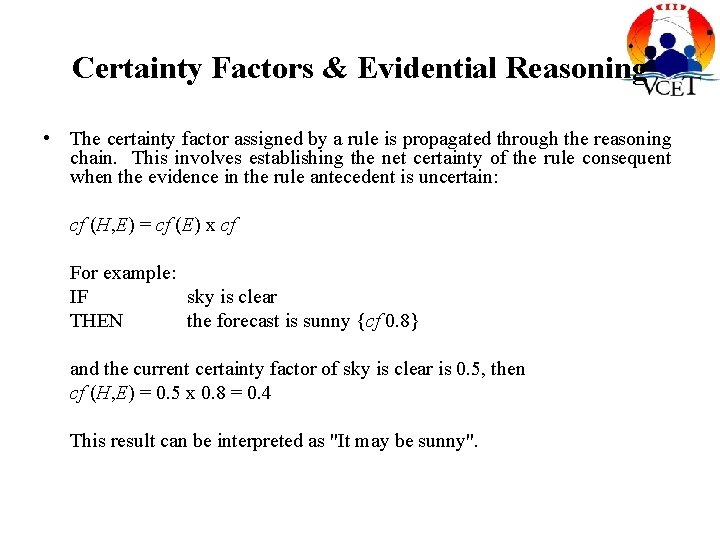Certainty Factors & Evidential Reasoning • The certainty factor assigned by a rule is propagated through the reasoning chain. This involves establishing the net certainty of the rule consequent when the evidence in the rule antecedent is uncertain: cf (H, E) = cf (E) x cf For example: IF sky is clear THEN the forecast is sunny {cf 0. 8} and the current certainty factor of sky is clear is 0. 5, then cf (H, E) = 0. 5 x 0. 8 = 0. 4 This result can be interpreted as "It may be sunny".Certainty Factors & Evidential Reasoning • For conjunctive rules such as: the certainty of hypothesis H, is established as follows: cf (H, E 1 E 2 . . . En) = min [cf (E 1), cf (E 2), . . . , cf (En)] x cf For example: IF AND THEN sky the forecast the action is clear is sunny is 'wear sunglasses' {cf 0. 8} and the certainty of sky is clear is 0. 9 and the certainty of the forecast of sunny is 0. 7, then cf (H, E 1 E 2) = min [0. 9, 0. 7] x 0. 8 = 0. 7 x 0. 8 = 0. 56Certainty Factors & Evidential Reasoning • For disjunctive rules such as: the certainty of hypothesis H, is established as follows: cf (H, E 1 E 2 . . . En) = max [cf (E 1), cf (E 2), . . . , cf (En)] x cf For example: IF OR THEN sky the forecast the action is overcast is rain is 'take an umbrella' {cf 0. 9} and the certainty of sky is overcast is 0. 6 and the certainty of the forecast of rain is 0. 8, then cf (H, E 1 E 2) = max [0. 6, 0. 8] x 0. 9 = 0. 8 x 0. 9 = 0. 72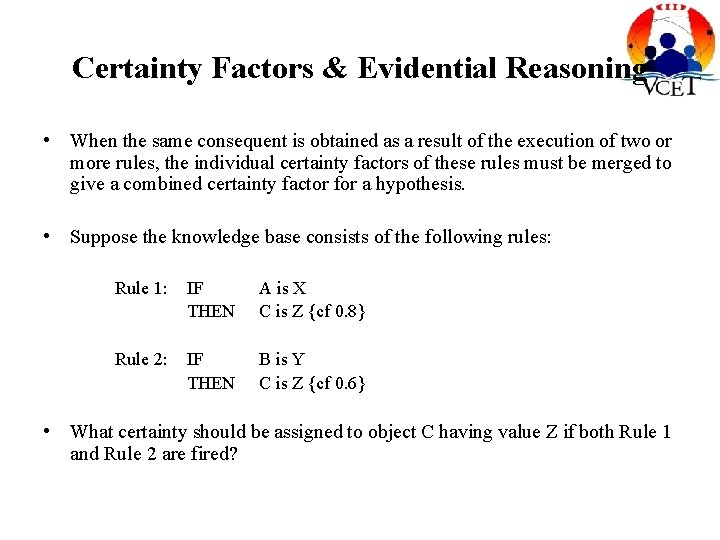Certainty Factors & Evidential Reasoning • When the same consequent is obtained as a result of the execution of two or more rules, the individual certainty factors of these rules must be merged to give a combined certainty factor for a hypothesis. • Suppose the knowledge base consists of the following rules: Rule 1: IF THEN A is X C is Z {cf 0. 8} Rule 2: IF THEN B is Y C is Z {cf 0. 6} • What certainty should be assigned to object C having value Z if both Rule 1 and Rule 2 are fired?Certainty Factors & Evidential Reasoning • Common sense suggests that, if we have two pieces of evidence (A is X and B is Y) from different sources (Rule 1 and Rule 2) supporting the same hypothesis (C is Z), then the confidence in this hypothesis should increase and become stronger than if only one piece of evidence had been obtained.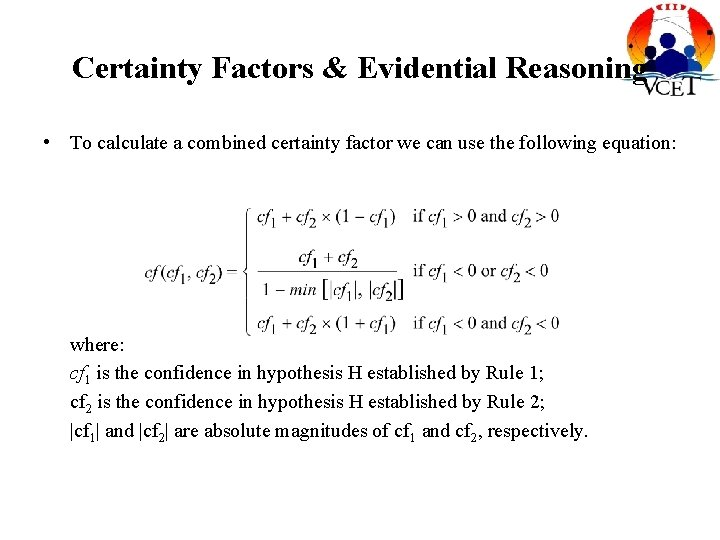Certainty Factors & Evidential Reasoning • To calculate a combined certainty factor we can use the following equation: where: cf 1 is the confidence in hypothesis H established by Rule 1; cf 2 is the confidence in hypothesis H established by Rule 2; |cf 1| and |cf 2| are absolute magnitudes of cf 1 and cf 2, respectively.Certainty Factors & Evidential Reasoning • The certainty factors theory provides a practical alternative to Bayesian reasoning. • The heuristic manner of combining certainty factors is different from the manner in which they would be combined if they were probabilities. • The certainty theory is not “mathematically pure” but does mimic the thinking process of a human expert.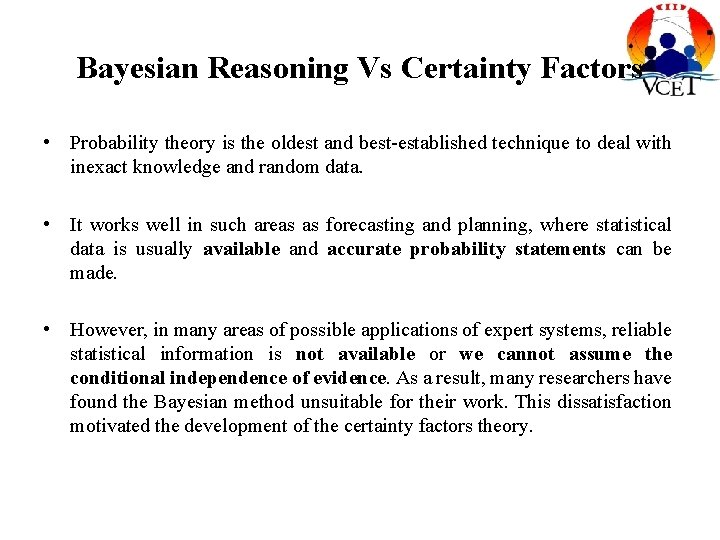Bayesian Reasoning Vs Certainty Factors • Probability theory is the oldest and best-established technique to deal with inexact knowledge and random data. • It works well in such areas as forecasting and planning, where statistical data is usually available and accurate probability statements can be made. • However, in many areas of possible applications of expert systems, reliable statistical information is not available or we cannot assume the conditional independence of evidence. As a result, many researchers have found the Bayesian method unsuitable for their work. This dissatisfaction motivated the development of the certainty factors theory.Bayesian Reasoning Vs Certainty Factors • Although the certainty factors approach lacks the mathematical correctness of the probability theory, it outperforms subjective Bayesian reasoning in such areas as diagnostics. • Certainty factors are used in cases where the probabilities are not known or are too difficult or expensive to obtain. The evidential reasoning mechanism can manage incrementally acquired evidence, the conjunction and disjunction of hypotheses, as well as evidences with different degrees of belief. • The certainty factors approach also provides better explanations of the control flow through a rule-based expert system.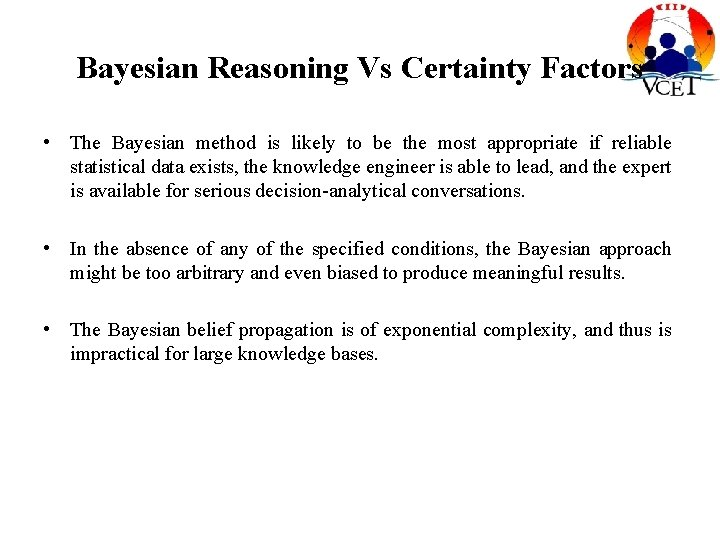Bayesian Reasoning Vs Certainty Factors • The Bayesian method is likely to be the most appropriate if reliable statistical data exists, the knowledge engineer is able to lead, and the expert is available for serious decision-analytical conversations. • In the absence of any of the specified conditions, the Bayesian approach might be too arbitrary and even biased to produce meaningful results. • The Bayesian belief propagation is of exponential complexity, and thus is impractical for large knowledge bases.